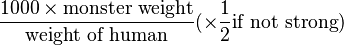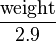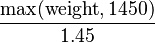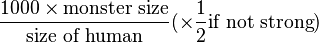# Monster carrying capacity

A monster's carrying capacity is calculated based on its weight or size relative to a human.

## Weighty monsters

If the monster has non-zero weight, its carrying capacity is$\frac{{1000 \times \text{monster weight}}}{\text{weight of human}} (\times \frac{1}{2} \text{if not strong})$.

The weight of a human is 1450 for this calculation. If a strong monster would have less than 1000 carrying capacity based on this calculation, then its carrying capacity is set to 1000.

This can be simplified to$\frac{\text{weight}}{2.9}$ if the monster is not strong, and$\frac{\text{max}(\text{weight}, 1450)}{1.45}$ if the monster is strong.

Some monsters have special rules for their carrying capacity:

## Weightless monsters

If the monster has zero weight, its carrying capacity is$\frac{1000 \times \text{monster size}}{\text{size of human}} (\times \frac{1}{2} \text{if not strong})$.

The numerical value used for monster size is that used for physical size: 0 for tiny, 1 for small, 2 for medium, 3 for large, 4 for huge, and 7 for gigantic. Humans have medium (2) size.